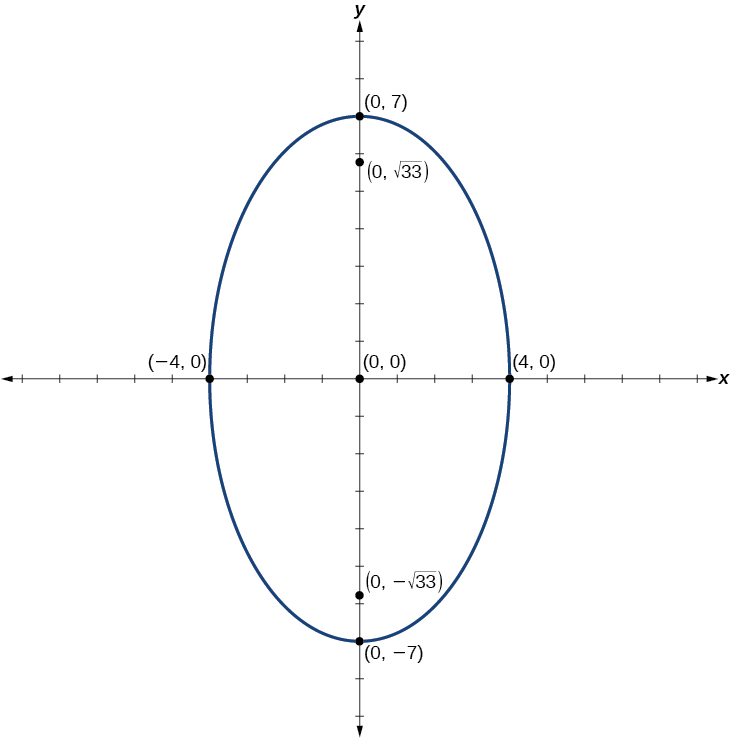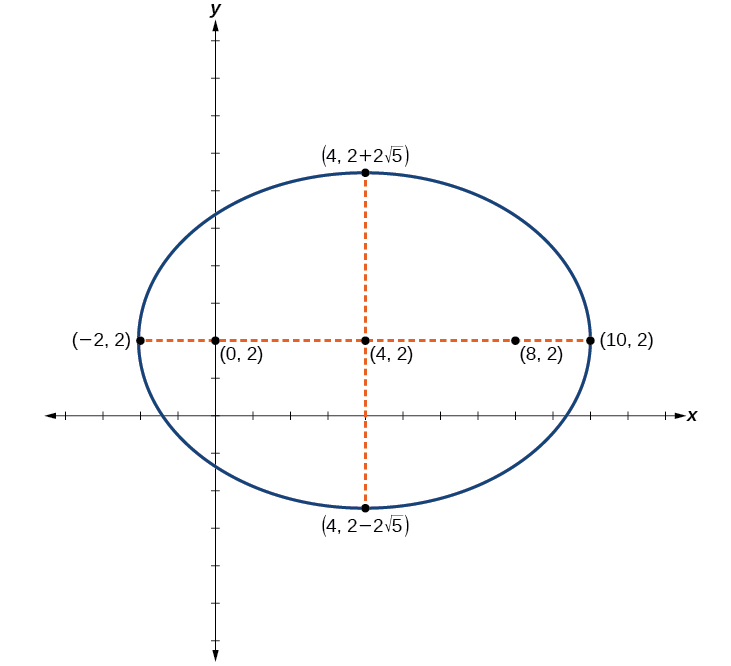# 12.1 The ellipse  (Page 6/16)

 Page 6 / 16

Graph the ellipse given by the equation $\text{\hspace{0.17em}}49{x}^{2}+16{y}^{2}=784.\text{\hspace{0.17em}}$ Rewrite the equation in standard form. Then identify and label the center, vertices, co-vertices, and foci.

Standard form: $\text{\hspace{0.17em}}\frac{{x}^{2}}{16}+\frac{{y}^{2}}{49}=1;\text{\hspace{0.17em}}$ center: $\text{\hspace{0.17em}}\left(0,0\right);\text{\hspace{0.17em}}$ vertices: $\text{\hspace{0.17em}}\left(0,±7\right);\text{\hspace{0.17em}}$ co-vertices: $\text{\hspace{0.17em}}\left(±4,0\right);\text{\hspace{0.17em}}$ foci: $\text{\hspace{0.17em}}\left(0,±\sqrt{33}\right)$## Graphing ellipses not centered at the origin

When an ellipse    is not centered at the origin, we can still use the standard forms to find the key features of the graph. When the ellipse is centered at some point, $\text{\hspace{0.17em}}\left(h,k\right),$ we use the standard forms for horizontal ellipses and for vertical ellipses. From these standard equations, we can easily determine the center, vertices, co-vertices, foci, and positions of the major and minor axes.

Given the standard form of an equation for an ellipse centered at $\text{\hspace{0.17em}}\left(h,k\right),$ sketch the graph.

1. Use the standard forms of the equations of an ellipse to determine the center, position of the major axis, vertices, co-vertices, and foci.
1. If the equation is in the form $\text{\hspace{0.17em}}\frac{{\left(x-h\right)}^{2}}{{a}^{2}}+\frac{{\left(y-k\right)}^{2}}{{b}^{2}}=1,\text{\hspace{0.17em}}$ where $\text{\hspace{0.17em}}a>b,\text{\hspace{0.17em}}$ then
• the center is $\text{\hspace{0.17em}}\left(h,k\right)$
• the major axis is parallel to the x -axis
• the coordinates of the vertices are $\text{\hspace{0.17em}}\left(h±a,k\right)$
• the coordinates of the co-vertices are $\text{\hspace{0.17em}}\left(h,k±b\right)$
• the coordinates of the foci are $\text{\hspace{0.17em}}\left(h±c,k\right)$
2. If the equation is in the form $\text{\hspace{0.17em}}\frac{{\left(x-h\right)}^{2}}{{b}^{2}}+\frac{{\left(y-k\right)}^{2}}{{a}^{2}}=1,\text{\hspace{0.17em}}$ where $\text{\hspace{0.17em}}a>b,\text{\hspace{0.17em}}$ then
• the center is $\text{\hspace{0.17em}}\left(h,k\right)$
• the major axis is parallel to the y -axis
• the coordinates of the vertices are $\text{\hspace{0.17em}}\left(h,k±a\right)$
• the coordinates of the co-vertices are $\text{\hspace{0.17em}}\left(h±b,k\right)$
• the coordinates of the foci are $\text{\hspace{0.17em}}\left(h,k±c\right)$
2. Solve for $\text{\hspace{0.17em}}c\text{\hspace{0.17em}}$ using the equation $\text{\hspace{0.17em}}{c}^{2}={a}^{2}-{b}^{2}.$
3. Plot the center, vertices, co-vertices, and foci in the coordinate plane, and draw a smooth curve to form the ellipse.

## Graphing an ellipse centered at ( h , k )

Graph the ellipse given by the equation, $\text{\hspace{0.17em}}\frac{{\left(x+2\right)}^{2}}{4}+\frac{{\left(y-5\right)}^{2}}{9}=1.\text{\hspace{0.17em}}$ Identify and label the center, vertices, co-vertices, and foci.

First, we determine the position of the major axis. Because $\text{\hspace{0.17em}}9>4,$ the major axis is parallel to the y -axis. Therefore, the equation is in the form $\text{\hspace{0.17em}}\frac{{\left(x-h\right)}^{2}}{{b}^{2}}+\frac{{\left(y-k\right)}^{2}}{{a}^{2}}=1,\text{\hspace{0.17em}}$ where $\text{\hspace{0.17em}}{b}^{2}=4\text{\hspace{0.17em}}$ and $\text{\hspace{0.17em}}{a}^{2}=9.\text{\hspace{0.17em}}$ It follows that:

• the center of the ellipse is $\text{\hspace{0.17em}}\left(h,k\right)=\left(-2,\text{5}\right)$
• the coordinates of the vertices are $\text{\hspace{0.17em}}\left(h,k±a\right)=\left(-2,5±\sqrt{9}\right)=\left(-2,5±3\right),$ or $\text{\hspace{0.17em}}\left(-2,\text{2}\right)\text{\hspace{0.17em}}$ and $\text{\hspace{0.17em}}\left(-2,\text{8}\right)$
• the coordinates of the co-vertices are $\text{\hspace{0.17em}}\left(h±b,k\right)=\left(-2±\sqrt{4},5\right)=\left(-2±2,5\right),$ or $\text{\hspace{0.17em}}\left(-4,5\right)\text{\hspace{0.17em}}$ and $\text{\hspace{0.17em}}\left(0,\text{5}\right)$
• the coordinates of the foci are $\text{\hspace{0.17em}}\left(h,k±c\right),\text{\hspace{0.17em}}$ where $\text{\hspace{0.17em}}{c}^{2}={a}^{2}-{b}^{2}.\text{\hspace{0.17em}}$ Solving for $\text{\hspace{0.17em}}c,$ we have:
$\begin{array}{l}\begin{array}{l}\\ c=±\sqrt{{a}^{2}-{b}^{2}}\end{array}\hfill \\ \text{\hspace{0.17em}}\text{\hspace{0.17em}}\text{\hspace{0.17em}}=±\sqrt{9-4}\hfill \\ \text{\hspace{0.17em}}\text{\hspace{0.17em}}\text{\hspace{0.17em}}=±\sqrt{5}\hfill \end{array}$

Therefore, the coordinates of the foci are $\text{\hspace{0.17em}}\left(-2,\text{5}-\sqrt{5}\right)\text{\hspace{0.17em}}$ and $\text{\hspace{0.17em}}\left(-2,\text{5+}\sqrt{5}\right).$

Next, we plot and label the center, vertices, co-vertices, and foci, and draw a smooth curve to form the ellipse.

Graph the ellipse given by the equation $\text{\hspace{0.17em}}\frac{{\left(x-4\right)}^{2}}{36}+\frac{{\left(y-2\right)}^{2}}{20}=1.\text{\hspace{0.17em}}$ Identify and label the center, vertices, co-vertices, and foci.

Center: $\text{\hspace{0.17em}}\left(4,2\right);\text{\hspace{0.17em}}$ vertices: $\text{\hspace{0.17em}}\left(-2,2\right)\text{\hspace{0.17em}}$ and $\text{\hspace{0.17em}}\left(10,2\right);\text{\hspace{0.17em}}$ co-vertices: $\text{\hspace{0.17em}}\left(4,2-2\sqrt{5}\right)\text{\hspace{0.17em}}$ and $\text{\hspace{0.17em}}\left(4,2+2\sqrt{5}\right);\text{\hspace{0.17em}}$ foci: $\text{\hspace{0.17em}}\left(0,2\right)\text{\hspace{0.17em}}$ and $\text{\hspace{0.17em}}\left(8,2\right)$Given the general form of an equation for an ellipse centered at ( h , k ), express the equation in standard form.

1. Recognize that an ellipse described by an equation in the form $\text{\hspace{0.17em}}a{x}^{2}+b{y}^{2}+cx+dy+e=0\text{\hspace{0.17em}}$ is in general form.
2. Rearrange the equation by grouping terms that contain the same variable. Move the constant term to the opposite side of the equation.
3. Factor out the coefficients of the $\text{\hspace{0.17em}}{x}^{2}\text{\hspace{0.17em}}$ and $\text{\hspace{0.17em}}{y}^{2}\text{\hspace{0.17em}}$ terms in preparation for completing the square.
4. Complete the square for each variable to rewrite the equation in the form of the sum of multiples of two binomials squared set equal to a constant, $\text{\hspace{0.17em}}{m}_{1}{\left(x-h\right)}^{2}+{m}_{2}{\left(y-k\right)}^{2}={m}_{3},$ where $\text{\hspace{0.17em}}{m}_{1},{m}_{2},$ and $\text{\hspace{0.17em}}{m}_{3}\text{\hspace{0.17em}}$ are constants.
5. Divide both sides of the equation by the constant term to express the equation in standard form.

what are you up to?
nothing up todat yet
Miranda
hi
jai
hello
jai
Miranda Drice
jai
aap konsi country se ho
jai
which language is that
Miranda
I am living in india
jai
good
Miranda
what is the formula for calculating algebraic
I think the formula for calculating algebraic is the statement of the equality of two expression stimulate by a set of addition, multiplication, soustraction, division, raising to a power and extraction of Root. U believe by having those in the equation you will be in measure to calculate it
Miranda
state and prove Cayley hamilton therom
hello
Propessor
hi
Miranda
the Cayley hamilton Theorem state if A is a square matrix and if f(x) is its characterics polynomial then f(x)=0 in another ways evey square matrix is a root of its chatacteristics polynomial.
Miranda
hi
jai
hi Miranda
jai
thanks
Propessor
welcome
jai
What is algebra
algebra is a branch of the mathematics to calculate expressions follow.
Miranda
Miranda Drice would you mind teaching me mathematics? I think you are really good at math. I'm not good at it. In fact I hate it. 😅😅😅
Jeffrey
lolll who told you I'm good at it
Miranda
something seems to wispher me to my ear that u are good at it. lol
Jeffrey
lolllll if you say so
Miranda
but seriously, Im really bad at math. And I hate it. But you see, I downloaded this app two months ago hoping to master it.
Jeffrey
which grade are you in though
Miranda
oh woww I understand
Miranda
Jeffrey
Jeffrey
Miranda
how come you finished in college and you don't like math though
Miranda
gotta practice, holmie
Steve
if you never use it you won't be able to appreciate it
Steve
I don't know why. But Im trying to like it.
Jeffrey
yes steve. you're right
Jeffrey
so you better
Miranda
what is the solution of the given equation?
which equation
Miranda
I dont know. lol
Jeffrey
Miranda
Jeffrey
answer and questions in exercise 11.2 sums
how do u calculate inequality of irrational number?
Alaba
give me an example
Chris
and I will walk you through it
Chris
cos (-z)= cos z .
cos(- z)=cos z
Mustafa
what is a algebra
(x+x)3=?
6x
Obed
what is the identity of 1-cos²5x equal to?
__john __05
Kishu
Hi
Abdel
hi
Ye
hi
Nokwanda
C'est comment
Abdel
Hi
Amanda
hello
SORIE
Hiiii
Chinni
hello
Ranjay
hi
ANSHU
hiiii
Chinni
h r u friends
Chinni
yes
Hassan
so is their any Genius in mathematics here let chat guys and get to know each other's
SORIE
I speak French
Abdel
okay no problem since we gather here and get to know each other
SORIE
hi im stupid at math and just wanna join here
Yaona
lol nahhh none of us here are stupid it's just that we have Fast, Medium, and slow learner bro but we all going to work things out together
SORIE
it's 12
what is the function of sine with respect of cosine , graphically
tangent bruh
Steve
cosx.cos2x.cos4x.cos8x
sinx sin2x is linearly dependent
what is a reciprocal
The reciprocal of a number is 1 divided by a number. eg the reciprocal of 10 is 1/10 which is 0.1
Shemmy
Reciprocal is a pair of numbers that, when multiplied together, equal to 1. Example; the reciprocal of 3 is ⅓, because 3 multiplied by ⅓ is equal to 1
Jeza
each term in a sequence below is five times the previous term what is the eighth term in the sequence
I don't understand how radicals works pls
How look for the general solution of a trig functionByByBy Jessica CollettBy Rohini AjayBy Brooke DelaneyBy Joli JuliannaBy Yacoub JayoghliBy Mistry BhaveshBy Tess ArmstrongBy OpenStaxBy OpenStaxBy Michael Sag